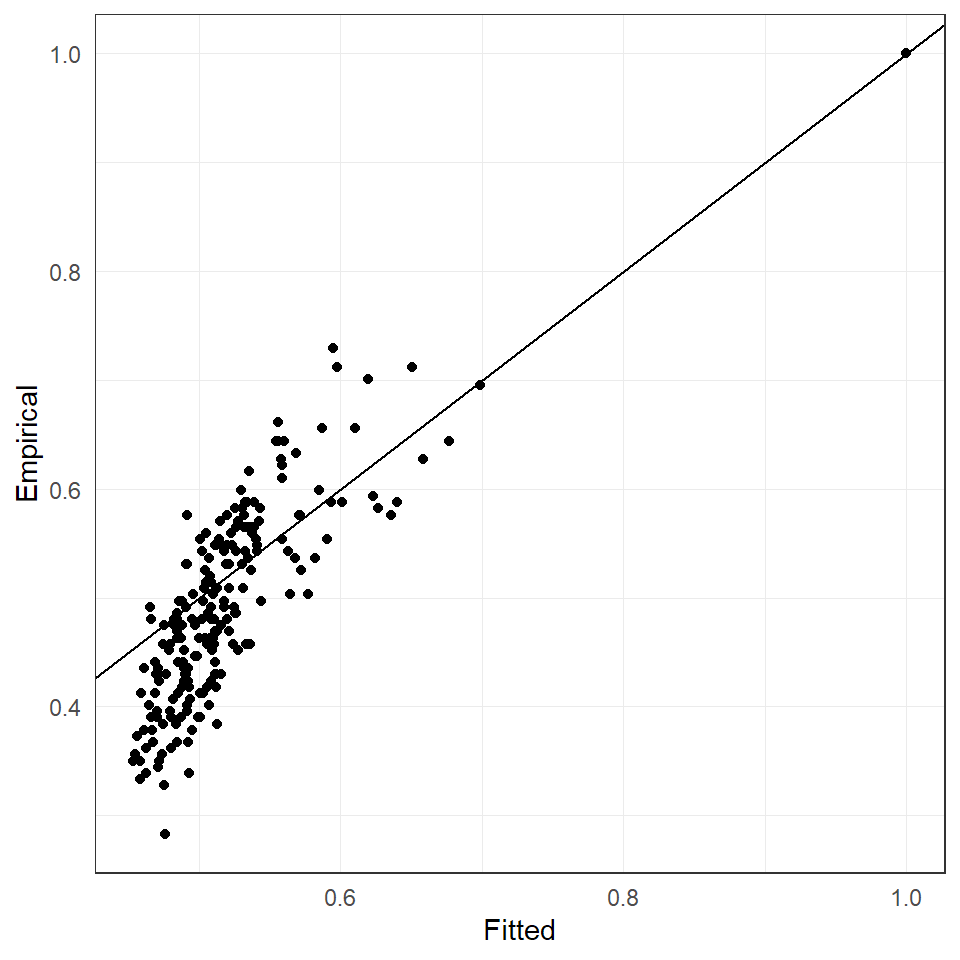library(graphicalExtremes)
library(ggplot2)
library(igraph)
theme_set(theme_bw() +
theme(plot.background = element_blank(),
legend.background = element_blank(),
strip.background = element_rect(fill = "white"),
plot.caption=element_text(size=7.5, hjust=0,
margin=margin(t=15)),
text = element_text(size = 11),
axis.ticks = element_blank(),
panel.grid.major = element_line(size = 0.25)))

The flights dataset contains daily total delays of major U.S. airlines. For details, see the corresponding documentation page. Below, we plot all airports in the dataset.

plotFlights(plotConnections = FALSE, map = "world", xyRatio = 2)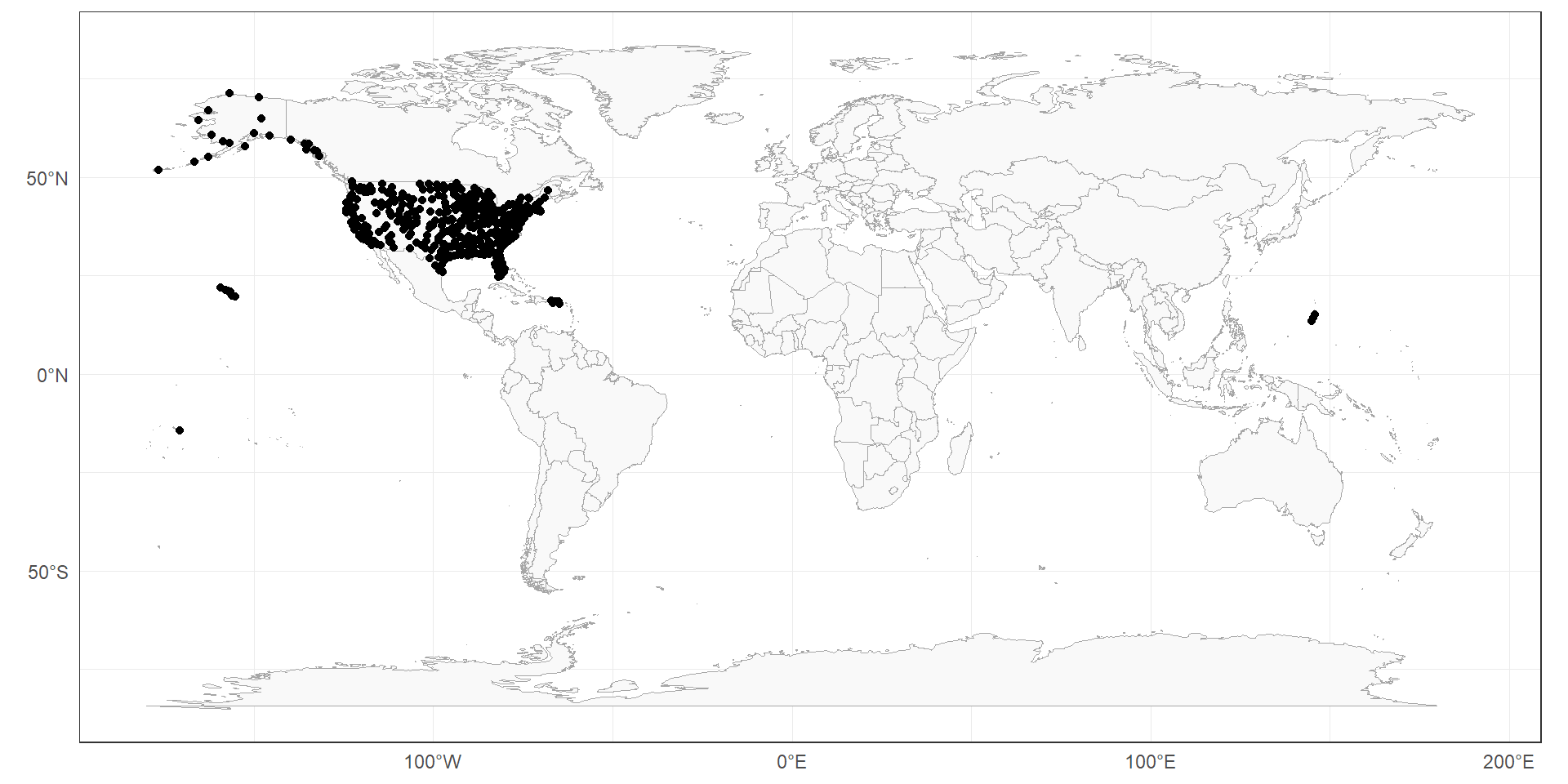To perform an example application, we consider the years 2010 - 2013 and choose (somewhat arbitrarily) airports from the westcoast with a large number of flights per year. Note: In the CRAN version of this package, these are the only available years. A version of this package with a larger dataset is available on GitHub

# Specify years
yearNames <- as.character(seq(2010, 2013))
minNFlights <- length(yearNames) * 1000

# Compute departures + arrivals per airport
flightsPerConnection <- apply(flights$flightCounts[, , yearNames], c(1, 2), sum) flightsPerAirport <- rowSums(flightsPerConnection) + colSums(flightsPerConnection) # Select airports (more than minNFlights and rough westcoast coordinates) ind <- ( flightsPerAirport >= minNFlights & flights$airports$Longitude < -119 & flights$airports$Longitude > -130 & flights$airports$Latitude > 25 & flights$airports$Latitude < 50 ) IATAs <- flights$airports$IATA[ind] # Plot airports + connections with at least monthly flights minNConnections <- length(yearNames) * 12 plotFlights( IATAs, minNFlights = minNConnections, useAirportNFlights = TRUE, useConnectionNFlights = TRUE )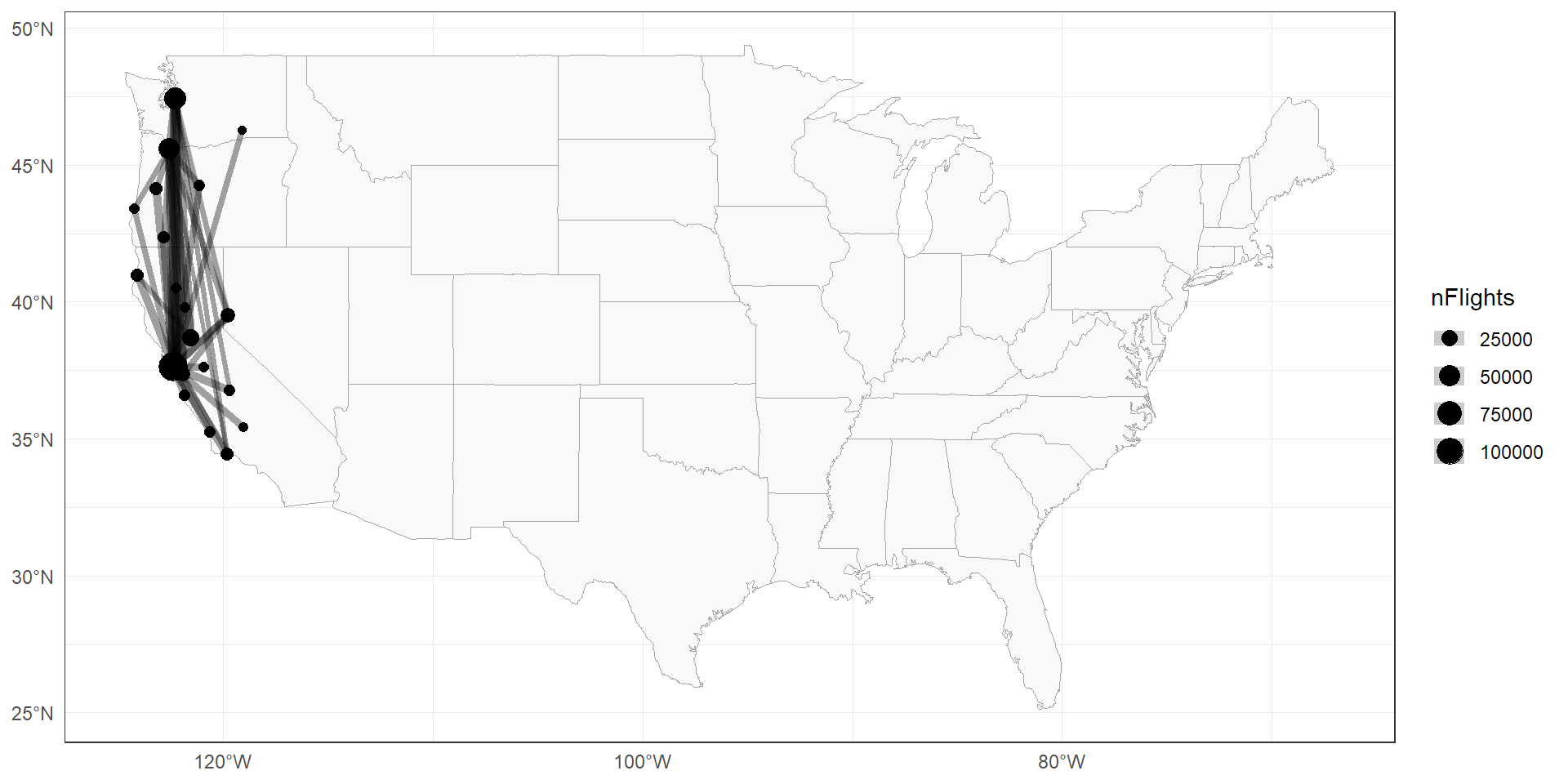Also, it is useful to create an igraph::graph() object for the flights connections. # Compute undirected flights per connection flightsPerConnectionUD <- flightsPerConnection + t(flightsPerConnection) # Consider only connections between selected airports flightsPerConnectionUD <- flightsPerConnectionUD[IATAs, IATAs] # Make flight graph A <- 1 * (flightsPerConnectionUD > minNConnections) flight_graph <- graph_from_adjacency_matrix(A, diag = FALSE, mode = "undirected") # Plot flight graph plotFlights( IATAs, graph = flight_graph, clipMap = 1.3, xyRatio = 1 )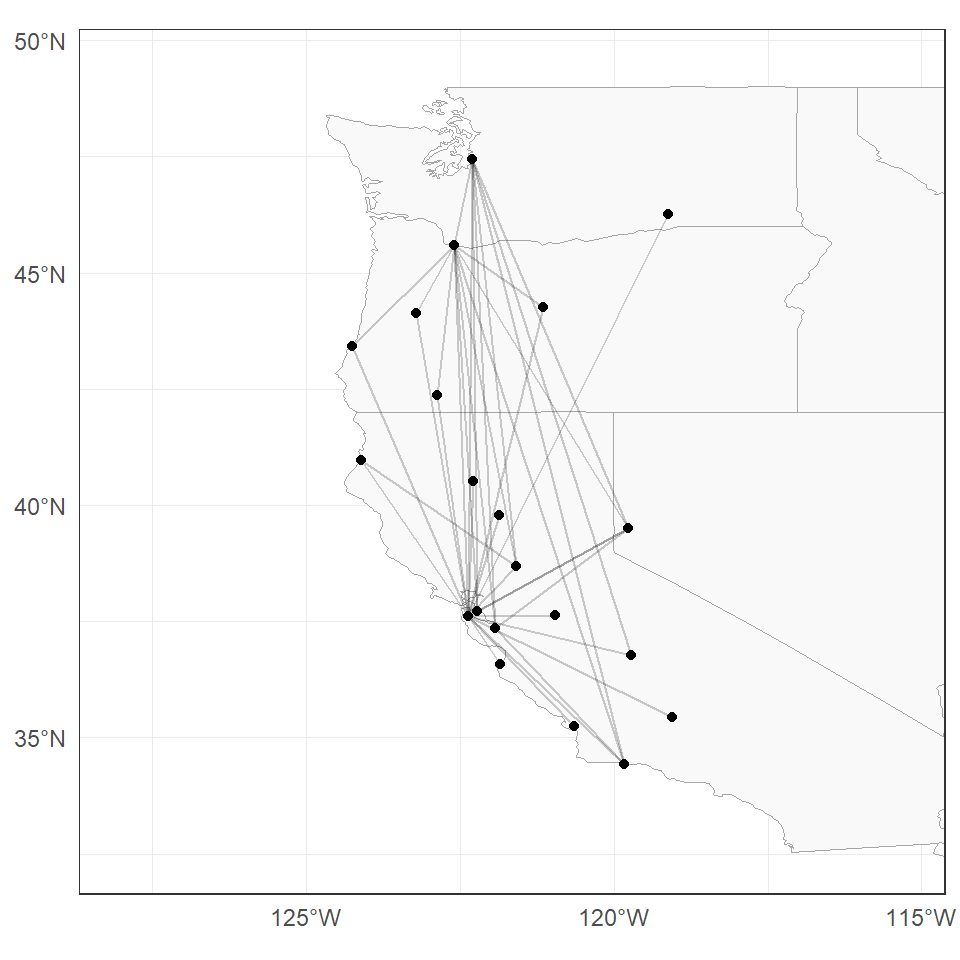# We use only departure delays, at selected airports, within the selected years dates <- as.Date(dimnames(flights$delays)[])
indDates <- format(dates, "%Y") %in% yearNames
mat <- flights$delays[indDates, IATAs, "departures"] # We remove all rows containing NAs rowHasNA <- apply(is.na(mat), 1, any) mat <- mat[!rowHasNA, ] # 1 Fitting a tree model 1. Fit an extremal tree model to the flight delays using emst(), choosing the threshold p = 0.7. p <- .7 flights_emst_fit <- emst(data = mat, p = p, method = "vario") 2. Plot the estimated tree on the US map and interpret the results. plotFlights( IATAs, graph = flights_emst_fit$graph,
xyRatio = 1,
clipMap = 1.3
)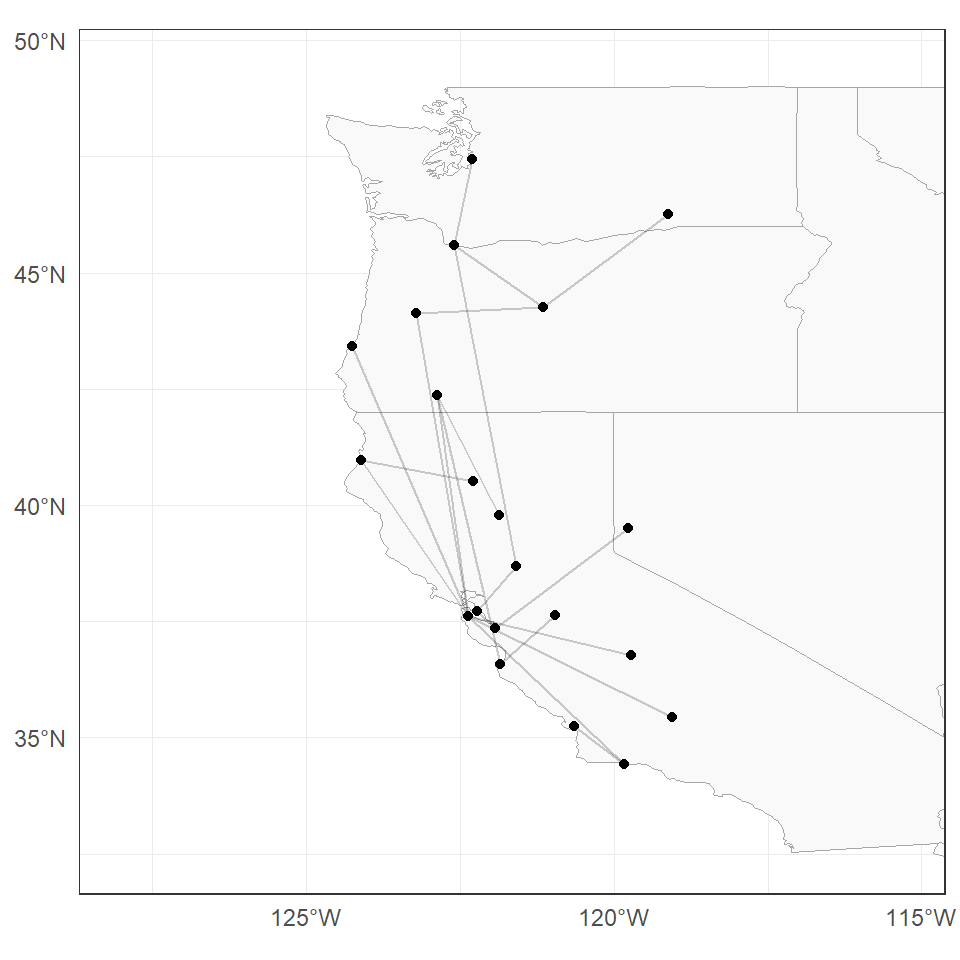3. Compute the BIC value of the fitted tree model.

flights_loglik_tree <- loglik_HR(
data = mat,
p = p,
Gamma = flights_emst_fit$Gamma, graph = flights_emst_fit$graph
)
cat("Tree BIC =", round(flights_loglik_tree, 2), "\n")
#> Tree BIC = 30837.64
4. Plot the empirical $$\chi$$ coefficient against the $$\chi$$ coefficient implied by the fitted model.

emp_chi_mat <- emp_chi(mat, p = p)

ggplot() +
geom_point(
aes(
x = c(Gamma2chi(flights_emst_fit$Gamma)), y = c(emp_chi_mat) ) ) + geom_abline(slope = 1, intercept = 0) + xlab("Fitted") + ylab("Empirical")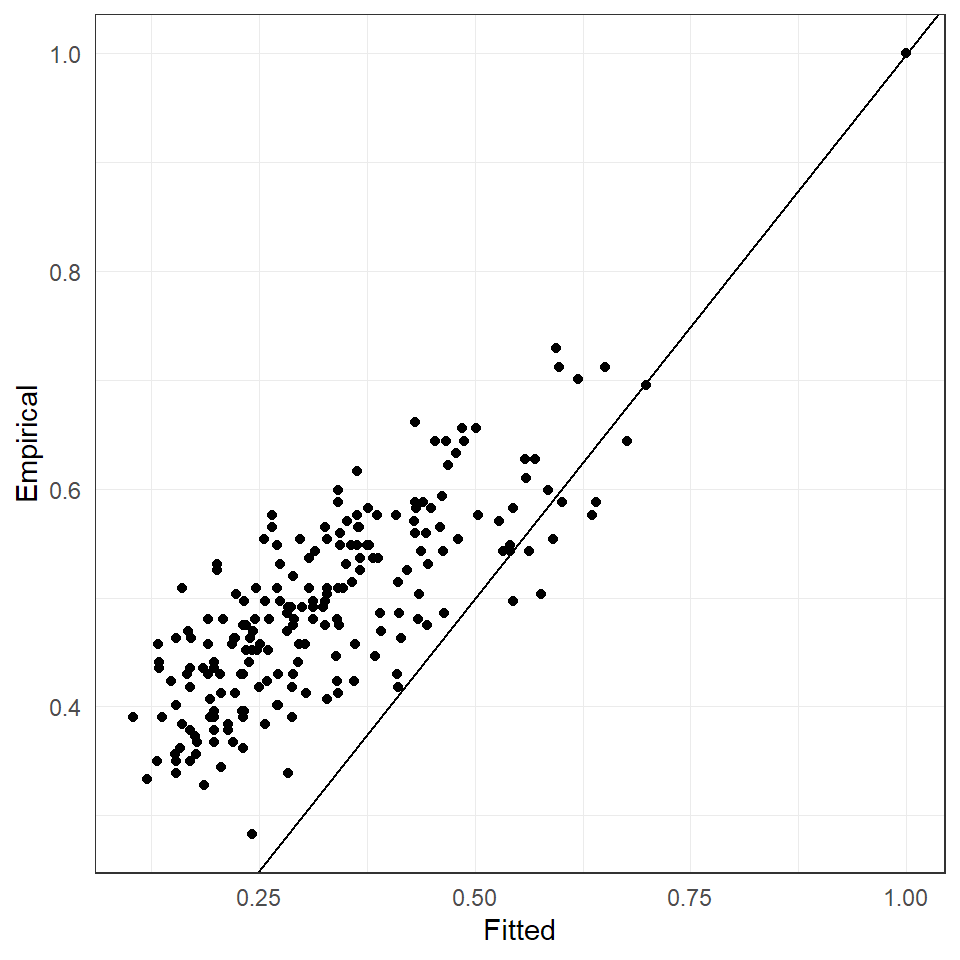5. Given the flight_graph object, fit a HR graphical model using fmpareto_graph_HR(). model_fit <- fmpareto_graph_HR( data = mat, graph = flight_graph, p = p, method = "vario" ) 6. Compute the BIC value for the flight_graph object and the corresponding Gamma matrix obtained at Step 5. flights_loglik_graph <- loglik_HR( data = mat, p = p, graph = flight_graph, Gamma = model_fit ) cat("BIC =", round(flights_loglik_graph, 2), "\n") #> BIC = 30156.53 7. Plot the empirical $$\chi$$ coefficient against the $$\chi$$ coefficient implied by the fitted model of Step 5. ggplot() + geom_point(aes( x = c(Gamma2chi(model_fit)), y = c(emp_chi_mat) )) + geom_abline(slope = 1, intercept = 0) + xlab("Fitted") + ylab("Empirical")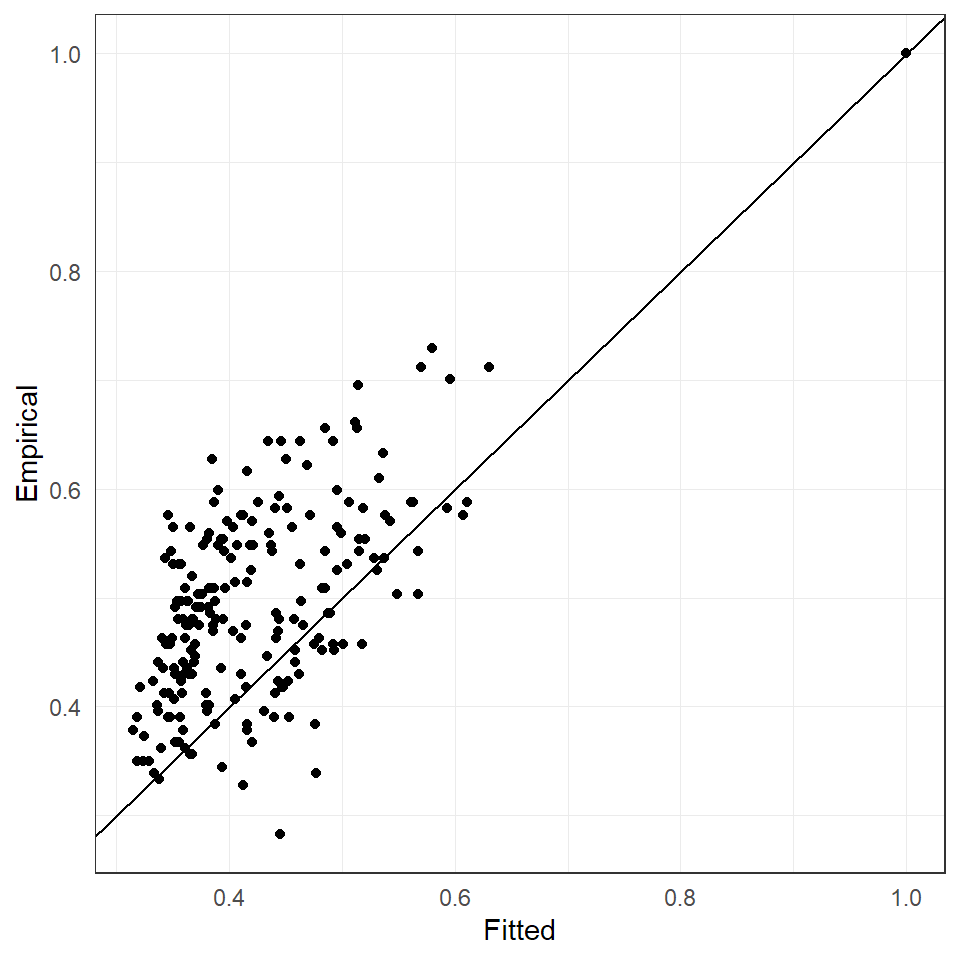# 2 Fitting a general model 1. Fit an extremal graphical lasso model with eglearn(), choosing threshold p = 0.7, and rholist = seq(1e-4, 0.10, length.out = 10). rholist <- seq(1e-4, 0.10, length.out = 10) flights_eglasso_fit <- eglearn(mat, p = p, rholist = rholist, complete_Gamma = TRUE) 2. Plot the estimated graph on the US map for different values of rho and interpret the results. plotFlights(IATAs, graph = flights_eglasso_fit$graph[], clipMap = 1.3, xyRatio = 1)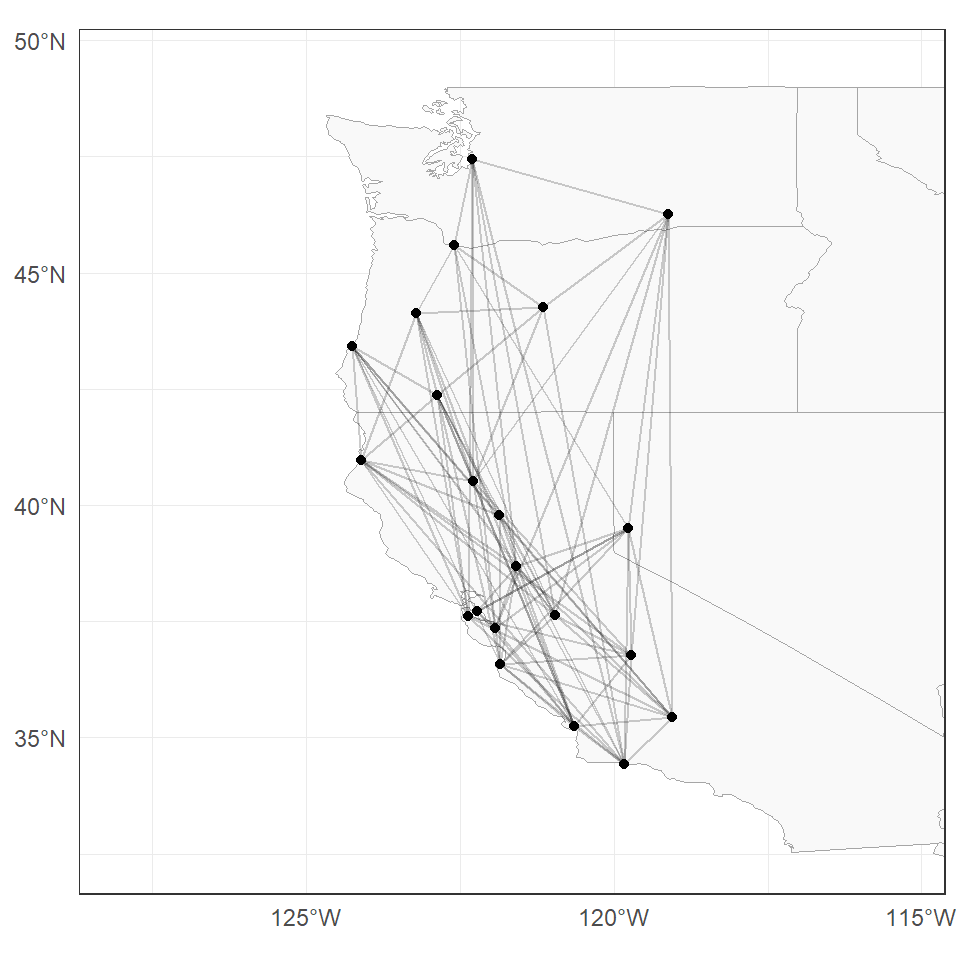3. Compute and plot the BIC values of the estimated models for different values of rho.

flights_loglik <- sapply(seq_along(rholist), FUN = function(j) {
loglik_HR(
data = mat, p = p,
Gamma = flights_eglasso_fit$Gamma[[j]], graph = flights_eglasso_fit$graph[[j]]
)
})

ggplot(mapping = aes(x = rholist, y = flights_loglik[3, ])) +
geom_line() +
geom_point(shape = 21, size = 3, stroke = 1, fill = "white") +
# geom_hline(aes(yintercept = flights_loglik_tree), lty = "dashed") +
xlab("rho") +
ylab("BIC") +
scale_x_continuous(
breaks = rholist,
labels = round(rholist, 3),
sec.axis = sec_axis(
trans = ~., breaks = rholist,
labels = sapply(
flights_eglasso_fit$graph, igraph::gsize ), name = "Number of edges" ) )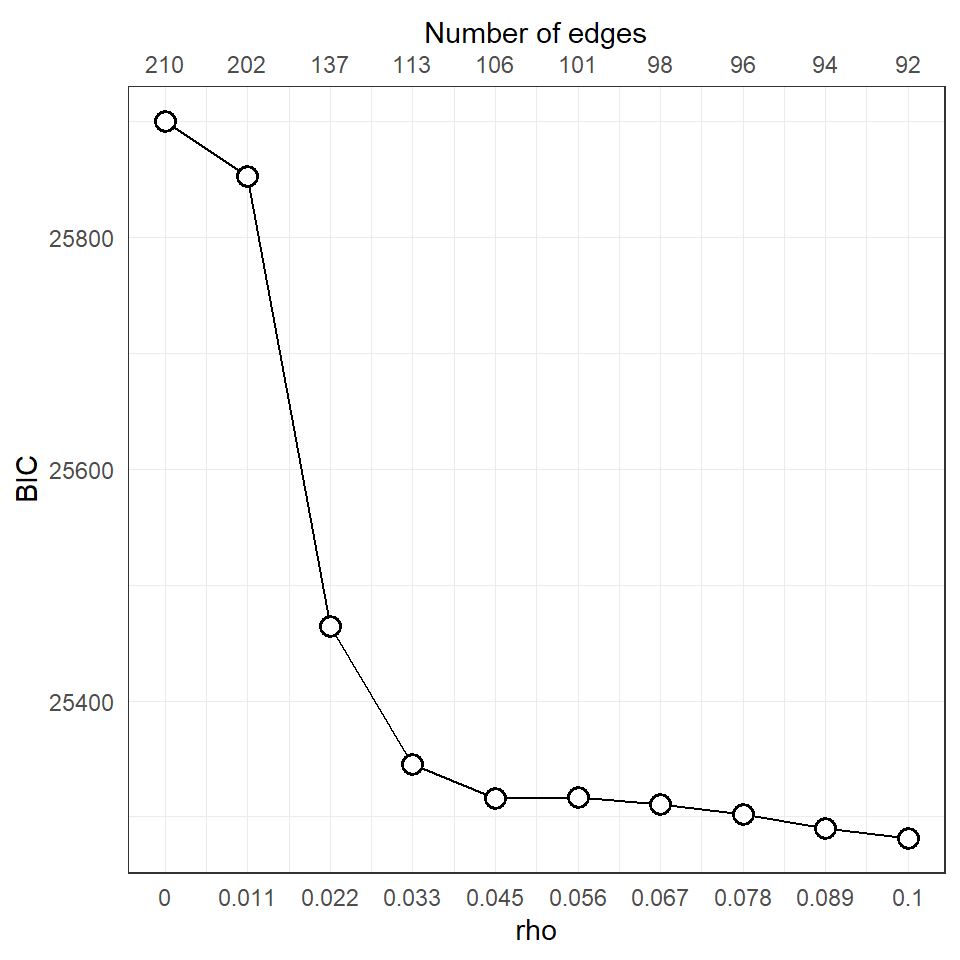4. Plot the $$\chi$$ coefficients implied by the best fitted model against the empirical $$\chi$$ coefficients. best_Gamma <- flights_eglasso_fit$Gamma[[which.min(flights_loglik[3,])]]

ggplot() +
geom_point(aes(x = c(Gamma2chi(best_Gamma)),
y = c(emp_chi_mat))) +
geom_abline(slope = 1, intercept = 0) +
xlab("Fitted") +
ylab("Empirical")